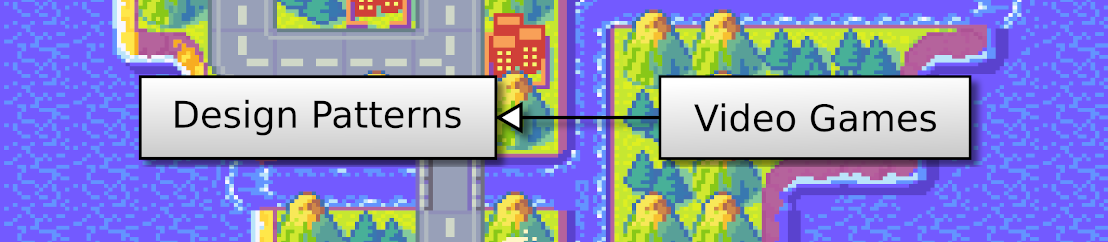## Discover Python and Patterns (13): Sprites

In this post, I propose to replace the rectangle in the previous program with a tank sprite, using a tileset.

This post is part of the Discover Python and Patterns series

## Tileset

I wish to create a "tank battle" game, so I looked for free game assets containing tank tilesets. You can found many on itch.io. I selected this one: zintoki.itch.io/ground-shaker, created by zintoki. I selected all the sprites I need and gathered them into a single image:

To load an image with Pygame, we can do as before with the `pygame.image.load()`function:

``unitsTexture = pygame.image.load("units.png")``

## Draw a sprite from a tileset

To draw a sprite from a tileset, we need to select the area in the tileset with the sprite, and copy it at any location on the screen:

Any instance of the `pygame.Surface` class can do this copy using the `blit()`method. We can obtain such a surface when we create a window:

``window = pygame.display.set_mode((256,256))``

This method has three arguments: the tileset image, the location in the surface (the screen in the case of the window), and the rectangle in the tileset.

We can represent locations using tuples `(x,y)`, but I will use instances of the `pygame.math.Vector2` class because it has many nice features:

``location = pygame.math.Vector2(x,y)``

Pygame represents rectangles with instances of the `pygame.Rect` class:

``rectangle = pygame.Rect(x,y,width,height)``

`x` and `y` are the coordinates of the top left corner and `width` and `height` the width and height of the rectangle.

Then, the `blit()` method can be used, for instance:

``window.blit(unitsTexture,location,rectangle)``

`location` is the location of the sprite in the surface (screen), and `rectangle` is the area in the `unitsTexture` tileset.

## Draw a tank sprite

For the case of our tank tileset, all sprites are `width = 64` per `height = 64` pixels. Furthermore, to select the tank in the first line and second column, the top left coordinates are: `x = 0` and `y = 64`. The rectangle is then:

``textureRect = pygame.Rect(64, 0, 64, 64)``

If we want to display the tank near the center of a window of 256 per 256 pixels, we need a rectangle at coordinates `x = 96` and `y = 96` (and with the same size):

``location = pygame.math.Vector2(96, 96)``

The following program contains all I presented (you can copy/paste it in Spyder, and run it):

``````import pygame

window = pygame.display.set_mode((256,256))
location = pygame.math.Vector2(96, 96)
rectangle = pygame.Rect(64, 0, 64, 64)
window.blit(unitsTexture,location,rectangle)

while True:
event = pygame.event.poll()
if event.type == pygame.QUIT:
break
pygame.display.update()

pygame.quit()``````

## Control the tank

I propose to replace the rectangle in the previous program to display and move a tank using the keyboard arrows.

Here is the structure of this program, updated from the previous one:

Class `UserInterface`:

• New attribute `cellSize`: defines the size of sprites. It makes a more readable code, and ease the update to sprites of a different size.
• New attribute `moveTankCommand`: it replaces the previous `moveCommandX` and `moveCommandY` attributes, and contains the next move command for the tank.
• New attribute `unitsTexture`: contains the tileset image

Class `GameState`:

• New attribute `worldSize`: defines the size of the world.
• New attribute `tankPos`: defines the location of the tank, and replaces the previous `x` and `y` attributes.

### Class `UserInterface` constructor

New lines are added to create new attributes:

``````def __init__(self):
pygame.init()

self.gameState = GameState()

self.cellSize = Vector2(64,64)

windowSize = self.gameState.worldSize.elementwise() * self.cellSize
self.window = pygame.display.set_mode((int(windowSize.x),int(windowSize.y)))
pygame.display.set_caption("Discover Python & Patterns - https://www.patternsgameprog.com")
self.moveTankCommand = Vector2(0,0)

self.clock = pygame.time.Clock()
self.running = True``````

As for most 2D coordinates, I use the `pygame.math.Vector2` class. To ease the reading, I added `from pygame.math import Vector2` at the beginning of the program, so we only have to type `Vector2` rather than `pygame.math.Vector2`.

I compute the size of the window according to the size of the game world (line 12). This expression is equivalent to:

``````windowSize = Vector2()
windowSize.x = self.gameState.worldSize.x * self.cellSize.x
windowSize.y = self.gameState.worldSize.y * self.cellSize.y``````

In other words, the size in pixels of the window is the size of the game world multiplied by the size of a cell/sprite. If the world size is (16,10) and the cell size (64,64), then the window size is `(16 * 64,10 * 64) = (1024,640)`.

Note that `Vector2` contains float values (numbers with a decimal part). So, to use it with `pygame.display.set_mode()`, we must convert it to a tuple of integers: `(int(windowSize.x),int(windowSize.y))`. If this expression is not clear, we could write:

``````windowSizeX = int(windowSize.x)
windowSizeY = int(windowSize.y)
windowSizeInteger = (windowSizeX,windowSizeY)
self.window = pygame.display.set_mode(windowSizeInteger)``````

### Class `UserInterface` method `render()`

This method draws a tank (only the base, no turret yet):

``````def render(self):
self.window.fill((0,0,0))

spritePoint = self.gameState.tankPos.elementwise()*self.cellSize
texturePoint = Vector2(1,0).elementwise()*self.cellSize
textureRect = Rect(int(texturePoint.x), int(texturePoint.y), int(self.cellSize.x),int(self.cellSize.y))
self.window.blit(self.unitsTexture,spritePoint,textureRect)

pygame.display.update()   ``````

The expression at line 4 is similar to the one I used to compute the size of the window: it multiplies the tank location by the size of a cell/sprite. This expression is equivalent to:

``````spritePoint = Vector2()
spritePoint.x = self.gameState.tankPos.x * self.cellSize.x
spritePoint.y = self.gameState.tankPos.y * self.cellSize.y``````

Line 5 computes the top-left corner of the rectangle that contains the tank sprite.

Line 6 creates a rectangle that contains the tank sprite. We have to convert float values into integers.

Line 7 draws the tank in the window surface.

### Class `GameState` method `update()`

The `update()` method works as before, except that the player can't move the tank outside the world:

``````def update(self,moveTankCommand):
self.tankPos += moveTankCommand

if self.tankPos.x < 0:
self.tankPos.x = 0
elif self.tankPos.x >= self.worldSize.x:
self.tankPos.x = self.worldSize.x - 1

if self.tankPos.y < 0:
self.tankPos.y = 0
elif self.tankPos.y >= self.worldSize.y:
self.tankPos.y = self.worldSize.y - 1``````